Contemporary Mathematics

# 1.3Understanding Venn Diagrams

Contemporary Mathematics1.3 Understanding Venn Diagrams

Figure 1.6 When assembling furniture, instructions with images are easier to follow, just like how set relationships are easier to understand when depicted graphically. (credit: "Time to assemble more Ikea furniture!" by Rod Herrea/Flickr, CC BY 2.0)

### Learning Objectives

After completing this section, you should be able to:

1. Utilize a universal set with two sets to interpret a Venn diagram.
2. Utilize a universal set with two sets to create a Venn diagram.
3. Determine the complement of a set.

Have you ever ordered a new dresser or bookcase that required assembly? When your package arrives you excitedly open it and spread out the pieces. Then you check the assembly guide and verify that you have all the parts required to assemble your new dresser. Now, the work begins. Luckily for you, the assembly guide includes step-by-step instructions with images that show you how to put together your product. If you are really lucky, the manufacturer may even provide a URL or QR code connecting you to an online video that demonstrates the complete assembly process. We can likely all agree that assembly instructions are much easier to follow when they include images or videos, rather than just written directions. The same goes for the relationships between sets.

### Interpreting Venn Diagrams

Venn diagrams are the graphical tools or pictures that we use to visualize and understand relationships between sets. Venn diagrams are named after the mathematician John Venn, who first popularized their use in the 1880s. When we use a Venn diagram to visualize the relationships between sets, the entire set of data under consideration is drawn as a rectangle, and subsets of this set are drawn as circles completely contained within the rectangle. The entire set of data under consideration is known as the universal set.

Consider the statement: All trees are plants. This statement expresses the relationship between the set of all plants and the set of all trees. Because every tree is a plant, the set of trees is a subset of the set of plants. To represent this relationship using a Venn diagram, the set of plants will be our universal set and the set of trees will be the subset. Recall that this relationship is expressed symbolically as: $Trees⊂Plants.Trees⊂Plants.$ To create a Venn diagram, first we draw a rectangle and label the universal set “$U=Plants.U=Plants.$” Then we draw a circle within the universal set and label it with the word “Trees.”

Figure 1.7

This section will introduce how to interpret and construct Venn diagrams. In future sections, as we expand our knowledge of relationships between sets, we will also develop our knowledge and use of Venn diagrams to explore how multiple sets can be combined to form new sets.

### Example 1.18

#### Interpreting the Relationship between Sets in a Venn Diagram

Write the relationship between the sets in the following Venn diagram, in words and symbolically.

Figure 1.8

1.
Write the relationship between the sets in the following Venn diagram, in words and symbolically.

So far, the only relationship we have been considering between two sets is the subset relationship, but sets can be related in other ways. Lions and tigers are both different types of cats, but no lions are tigers, and no tigers are lions. Because the set of all lions and the set of all tigers do not have any members in common, we call these two sets disjoint sets, or non-overlapping sets.

Two sets $AA$ and $BB$ are disjoint sets if they do not share any elements in common. That is, if $aa$ is a member of set $AA$, then $aa$ is not a member of set $BB$. If $bb$ is a member of set $BB$, then $bb$ is not a member of set $AA$. To represent the relationship between the set of all cats and the sets of lions and tigers using a Venn diagram, we draw the universal set of cats as a rectangle and then draw a circle for the set of lions and a separate circle for the set of tigers within the rectangle, ensuring that the two circles representing the set of lions and the set of tigers do not touch or overlap in any way.

Figure 1.9

### Example 1.19

#### Describing the Relationship between Sets

Describe the relationship between the sets in the following Venn diagram.

Figure 1.10

1.

Describe the relationship between the sets in the following Venn diagram.

### Creating Venn Diagrams

The main purpose of a Venn diagram is to help you visualize the relationship between sets. As such, it is necessary to be able to draw Venn diagrams from a written or symbolic description of the relationship between sets.

#### Procedure

To create a Venn diagram:

1. Draw a rectangle to represent the universal set, and label it $U=set nameU=set name$.
2. Draw a circle within the rectangle to represent a subset of the universal set and label it with the set name.

### Checkpoint

If there are multiple disjoint subsets of the universal set, their separate circles should not touch or overlap.

### Example 1.20

#### Drawing a Venn Diagram to Represent the Relationship Between Two Sets

Draw a Venn diagram to represent the relationship between each of the sets.

1. All rectangles are parallelograms.
2. All women are people.

1.
Draw a Venn diagram to represent the relationship between each of the sets. All natural numbers are integers.
2.
$A \subset U$. Draw a Venn diagram to represent this relationship.

### Example 1.21

#### Drawing a Venn Diagram to Represent the Relationship Between Three Sets

All bicycles and all cars have wheels, but no bicycle is a car. Draw a Venn diagram to represent this relationship.

1.
Airplanes and birds can fly, but no birds are airplanes. Draw a Venn diagram to represent this relationship.

### The Complement of a Set

Recall that if set $AA$ is a proper subset of set $UU$, the universal set (written symbolically as $A⊂UA⊂U$), then there is at least one element in set $UU$ that is not in set $AA$. The set of all the elements in the universal set $UU$ that are not in the subset $AA$ is called the complement of set $AA$, $A'A'$. In set builder notation this is written symbolically as: $A'={x∈U|x∉A}.A'={x∈U|x∉A}.$ The symbol $∈∈$ is used to represent the phrase, “is a member of,” and the symbol $∉∉$ is used to represent the phrase, “is not a member of.” In the Venn diagram below, the complement of set $AA$ is the region that lies outside the circle and inside the rectangle. The universal set $UU$ includes all of the elements in set $AA$ and all of the elements in the complement of set $AA$, and nothing else.

Figure 1.16

Consider the set of digit numbers. Let this be our universal set, $U={0,1,2,3,4,5,6,7,8,9}.U={0,1,2,3,4,5,6,7,8,9}.$ Now, let set $AA$ be the subset of $UU$ consisting of all the prime numbers in set $UU$, $A={2,3,5,7}.A={2,3,5,7}.$ The complement of set $AA$ is $A'={0,1,4,6,8,9}.A'={0,1,4,6,8,9}.$ The following Venn diagram represents this relationship graphically.

Figure 1.17

### Example 1.22

#### Finding the Complement of a Set

For both of the questions below, $AA$ is a proper subset of $UU$.

1. Given the universal set $U={Billie Eilish, Donald Glover, Bruno Mars, Adele, Ed Sheeran}U={Billie Eilish, Donald Glover, Bruno Mars, Adele, Ed Sheeran}$ and set $A={Donald Glover, Bruno Mars, Ed Sheeran}A={Donald Glover, Bruno Mars, Ed Sheeran}$, find $A'.A'.$
2. Given the universal set $U={d|d is a dog}U={d|d is a dog}$ and $B={b ∈ U|b is a beagle}B={b ∈ U|b is a beagle}$, find $B'.B'.$

For both of the questions below, $A$ is a proper subset of $U$.
1.
Given the universal set $U = \left\{ {{\text{red, orange, yellow, green, blue, indigo, violet}}} \right\}$ and set $A = \left\{ {{\text{yellow, red, blue}}} \right\}$, find $A'$.
2.
Given the universal set $U = \{ c|c\,{\text{is a cat\} }}$ and set $A = \{ c \in U|c{\text{ is not a lion\}, }}$ find $A'$.

15.
A Venn diagram is a graphical representation of the _____________ between sets.
16.
In a Venn diagram, the set of all data under consideration, the _____________ set, is drawn as a rectangle.
17.
Two sets that do not share any elements in common are _____________ sets.
18.
The _____________ of a subset $A$ or the universal set, $U$, is the set of all members of $U$ that are not in $A$.
19.
The sets $A$ and $A'$ are _____________ subsets of the universal set.

### Section 1.3 Exercises

For the following exercises, interpret each Venn diagram and describe the relationship between the sets, symbolically and in words.
1 .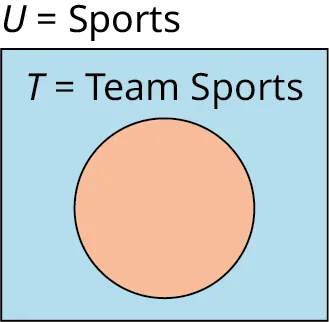2 .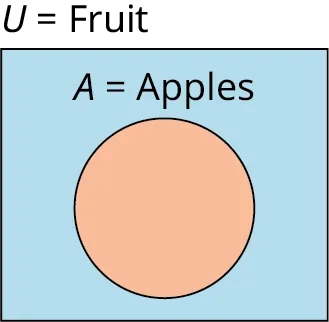3 .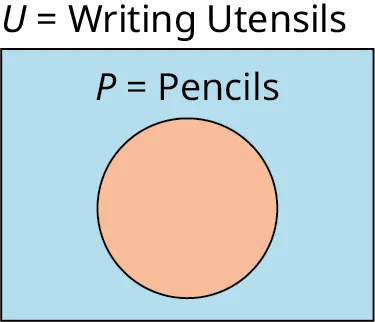4 .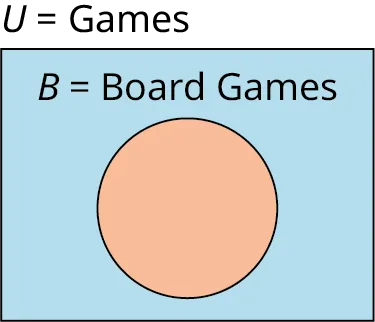5 .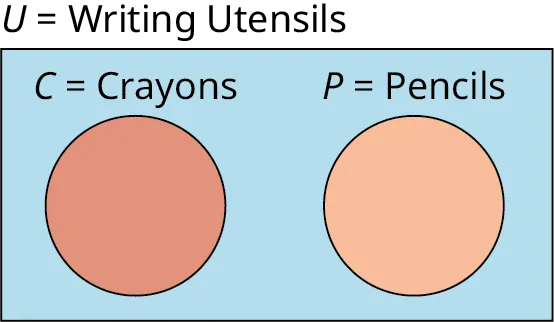6 .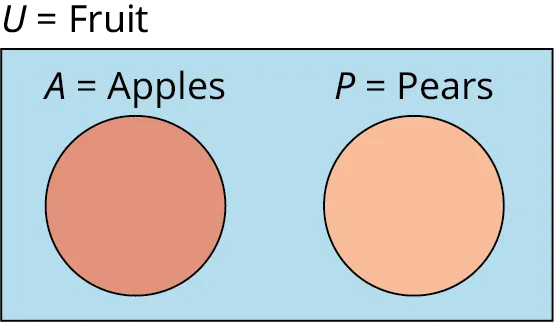7 .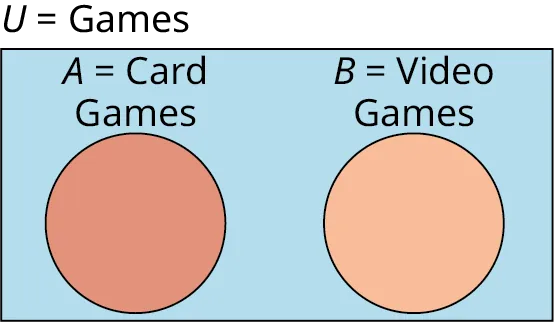8 .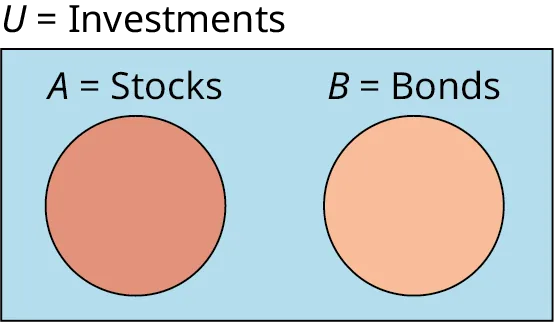For the following exercises, create a Venn diagram to represent the relationships between the sets.
9 .
All birds have wings.
10 .
All cats are animals.
11 .
All almonds are nuts, and all pecans are nuts, but no almonds are pecans.
12 .
All rectangles are quadrilaterals, and all trapezoids are quadrilaterals, but no rectangles are trapezoids.
13 .
Lizards $\subset$ Reptiles.
14 .
Ladybugs $\subset$ Insects.
15 .
Ladybugs $\subset$ Insects and Ants $\subset$ Insects, but no Ants are Ladybugs.
16 .
Lizards $\subset$ Reptiles and Snakes $\subset$ Reptiles, but no Lizards are Snakes.
17 .
$A$ and $B$ are disjoint subsets of $U.$
18 .
$C$ and $D$ are disjoint subsets of $U$.
19 .
$T$ is a subset of $U$.
20 .
$S$ is a subset of $U$.
21 .
$J =$ Jazz, $M =$ Music, and $J \subset M$.
22 .
$R =$ Reggae, $M =$ Music, and $R \subset M$.
23 .
$J =$ Jazz, $R =$ Reggae, and $M =$ Music are sets with the following relationships: $J \subset M,$ $R \subset M,$ and $R$ is disjoint from $J$.
24 .
$J =$ Jazz, $B =$ Bebop, and $M =$ Music are sets with the following relationships: $J \subset M$ and $B \subset J$.
For the following exercises, the universal set is the set of single digit numbers, $U = \{ 0,1,2,3,4,5,6,7,8,9\}$ . Find the complement of each subset of $U$.
25 .
$A = \{ 6,7,8\}$
26 .
$A = \{ 0,2,4,6,8\}$
27 .
$A = \{ {\text{ }}\}$
28 .
$A = \{ 0,1,4,6,8,9\}$
29 .
$A = \{ 0,1,2,3,4,5,6,7,8,9\}$
30 .
$A = \{ 0,1,3,4,5,6,7,9\}$
31 .
$A = \{ 1,2,3,4,5,6,7,8,9\}$
32 .
$A = \{ 0,3,6,9\}$
For the following exercises, the universal set is $U =$ {Bashful, Doc, Dopey, Grumpy, Happy, Sleepy, Sneezy}. Find the complement of each subset of $U$.
33 .
$A =$ {Happy, Bashful, Grumpy}
34 .
$A =$ {Sleepy, Sneezy}
35 .
$A =$ {Doc}
36 .
$A =$ {Doc, Dopey}
37 .
$A = \emptyset$
38 .
$A =$ {Doc, Grumpy, Happy, Sleepy, Bashful, Sneezy, Dopey}
For the following exercises, the universal set is $U = \mathbb{N} = \{ 1,2,3, \ldots \}$ . Find the complement of each subset of $U$.
39 .
$A = \{ 1,2,3,4,5\}$
40 .
$A = \{ 1,3,5, \ldots \}$
41 .
$A = \{ 1\}$
42 .
$A = \{ 4,5,6, \ldots \}$
For the following exercises, use the Venn diagram to determine the members of the complement of set $A,{\text{ }}A'$.
43 .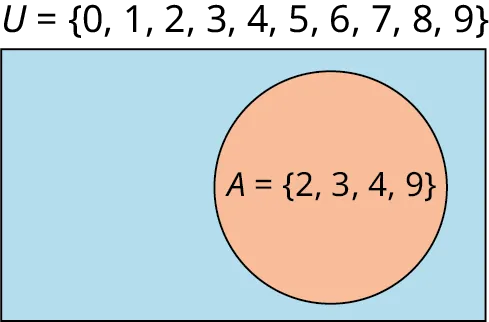44 .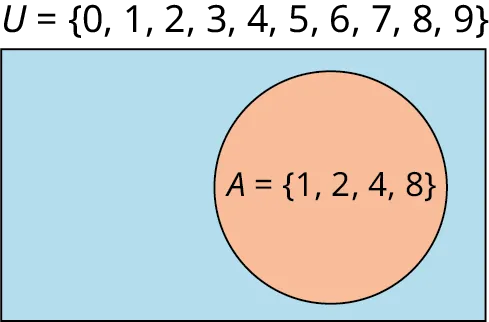45 .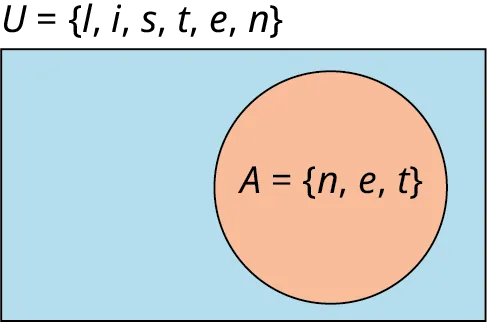46 .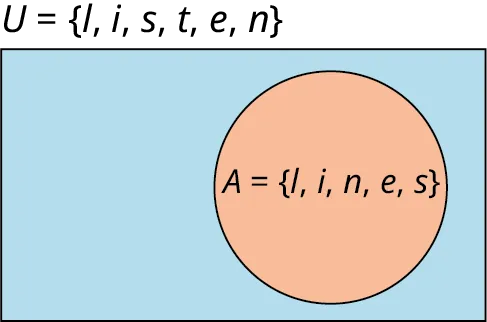Order a print copy

As an Amazon Associate we earn from qualifying purchases.